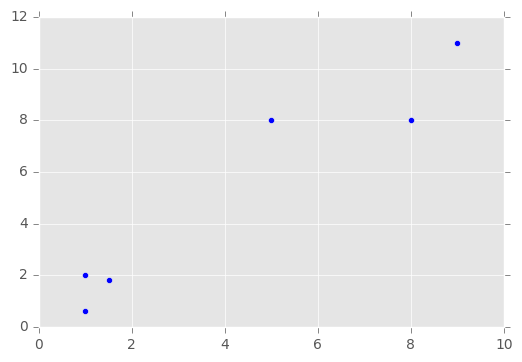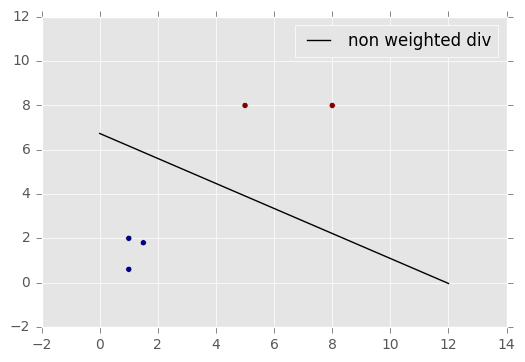# Python examples and notes for Machine Learning for Computational Linguistics¶

(C) 2017-2019 by Damir Cavar

Download: This and various other Jupyter notebooks are available from my GitHub repo.

Version: 1.1, September 2019

This is a tutorial related to the discussion of SpamAssassin in the textbook Machine Learning: The Art and Science of Algorithms that Make Sense of Data by Peter Flach.

This tutorial was developed as part of my course material for the course Machine Learning for Computational Linguistics in the Computational Linguistics Program of the Department of Linguistics at Indiana University.

## SpamAssassin¶

The linear classifier can be described as follows. A test $x$ returns $1$ (for true), if it succedes, otherwise it returns $0$. The $i^{th}$ test in the set of tests $x$ is refered to as $x_i$. The weight of the $i^{th}$ test is denoted as $w_i$.

The total score of test results for a specific e-mail can be expressed as the sum of the products of $n$ test results and corresponding weights, that is $\sum_{i=1}^{n} w_i x_i$.

If we assume two tests $x_1$ and $x_2$ with the corresponding weights $w_1 = 4$ and $w_2 = 4$, for some e-mail $e_1$ the tests could result in two positives $x_1 = 1$ and $x_2 = 1$. The computation of the equation above for the results can be coded in Python in the following way:

In :
x = (1, 0)
w = (4, 4)

result = 0
for e in range(len(x)):
result += x[e] * w[e]

print(result)

4


If we specify a threshold $t$ that seperates spam from ham, with $t = 5$ in our example, the decision for spam or ham could be coded in Python as:

In :
t = 5

if result >= t:
print("spam", result)
else:
print("ham", result)

ham 4


In the code example above we define $x$ and $w$ as vectors of the same length. The computation of the result could be achieved even easier by making use of linear algebra and calculating the dot-product of $x$ and $w$:

In :
import numpy

wn = [4, 4]
xn = [1, 1]

numpy.dot(wn, xn)

Out:
8

We can use a trick to manipulate the data to be rooted in the origin of an extended coordiante system. We can add a new dimension by adding a new virtual test result $x_0 = 1$ and a corresponding weight $w_0 = -t$. This way the decision boundary $t$ can be moved to $0$:

In :
x0 = (1, 1, 1)
w0 = (-t, 4, 4)

numpy.dot(w0, x0)

Out:
3

This kind of transformation of the vector space is usefull for other purposes as well. More on that later.

## Creating and Using an SVM Classifier¶

The following example is inspired and partially taken from the page Linear SVC Machine learning SVM example with Python.

To start learning and classifying, we will need to import some Python modules in addition to the ones above:

In :
import matplotlib.pyplot
from matplotlib import style

style.use("ggplot")

from sklearn import svm


We will use two features that represent the axis on a graph. The samples are tuples taken from the ordered arrays $x$ and $y$, that is, the $i^{th}$ sample is $X_i = (x_i, y_i)$, sample $X_1 = (1,2)$, sample $X_2 = (5, 8)$, and so on.

In :
x = [1, 5, 1.5, 8, 1, 9]
y = [2, 8, 1.8, 8, 0.6, 11]


We can plot the datapoints now:

In :
matplotlib.pyplot.scatter(x,y)
matplotlib.pyplot.show()We can create an array of features, that is, we convert the coordinates in the $x$ and $y$ feature arrays above to an array of tuples that represent the datapoints or features of samples:

In :
X = numpy.array([[1,2],
[5,8],
[1.5,1.8],
[8,8],
[1,0.6],
[9,11]])


Assuming two classes represented by $0$ and $1$, we can encode the assignment of the datapoints in $X$ to classes $0$ or $1$ by using a vector with the class labels in the order of the samples in $X$. The $i^{th}$ datapoint of $X$ is assigned to the $i^{th}$ class label in $y$.

In :
y = [0,1,0,1,0,1]


We define a classifier as a linear Support Vector Classifier using the svm module of Scikit-learn:

In :
classifier = svm.SVC(kernel='linear')


We train the classifier using our features in X and the labels in y:

In :
classifier.fit(X,y)

Out:
SVC(C=1.0, cache_size=200, class_weight=None, coef0=0.0,
decision_function_shape=None, degree=3, gamma='auto', kernel='linear',
max_iter=-1, probability=False, random_state=None, shrinking=True,
tol=0.001, verbose=False)

We can now create a new sample and ask the classifier for a guess to which class this sample belongs. Note that in the following code we generate a numpy array from the features $[0.58, 0.76]$. This array needs to be reshaped to an array the contains one element, an array with a set of sample features.

In :
sample = numpy.array([0.58,0.76]).reshape(1,-1)

print("Sample:", sample)

print(" Class:", classifier.predict(sample))

Sample: [[ 0.58  0.76]]
Class: 


Instead of using the reshape() function, we could have also defined the sample directly as an array with a sample feature array:

In :
sample = numpy.array( [ [0.58,0.76] ] )


The following code will visualize the data and the identified hyperplane that separates the two classes.

In :
w = classifier.coef_
print(w)

a = -w / w

xx = numpy.linspace(0,12)
yy = a * xx - classifier.intercept_ / w

h0 = matplotlib.pyplot.plot(xx, yy, 'k-', label="non weighted div")

matplotlib.pyplot.scatter(X[:, 0], X[:, 1], c = y)
matplotlib.pyplot.legend()
matplotlib.pyplot.show()

[ 0.1380943   0.24462418](C) 2017-2019 by Damir Cavar - Creative Commons Attribution-ShareAlike 4.0 International License (CA BY-SA 4.0); portions taken from the referenced sources.